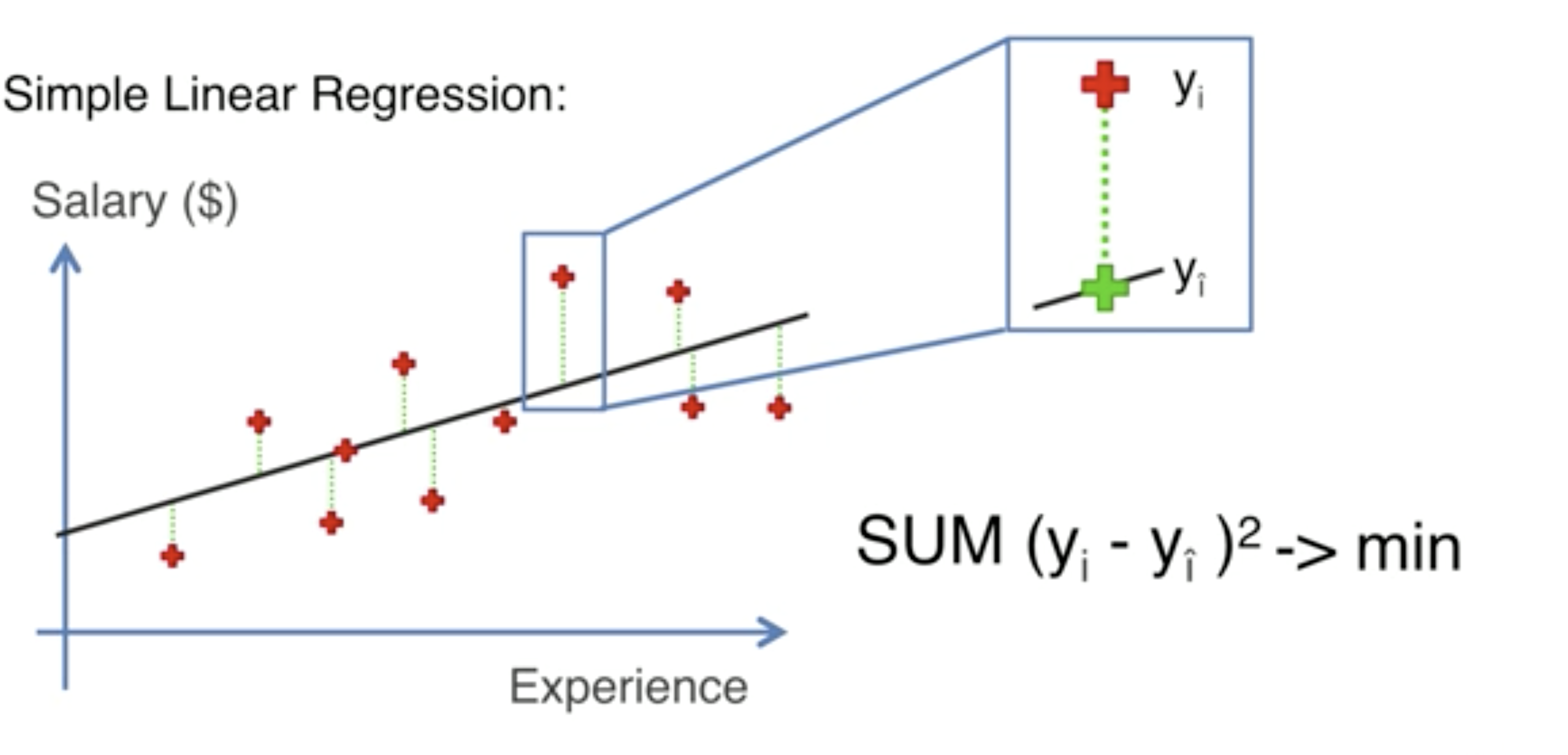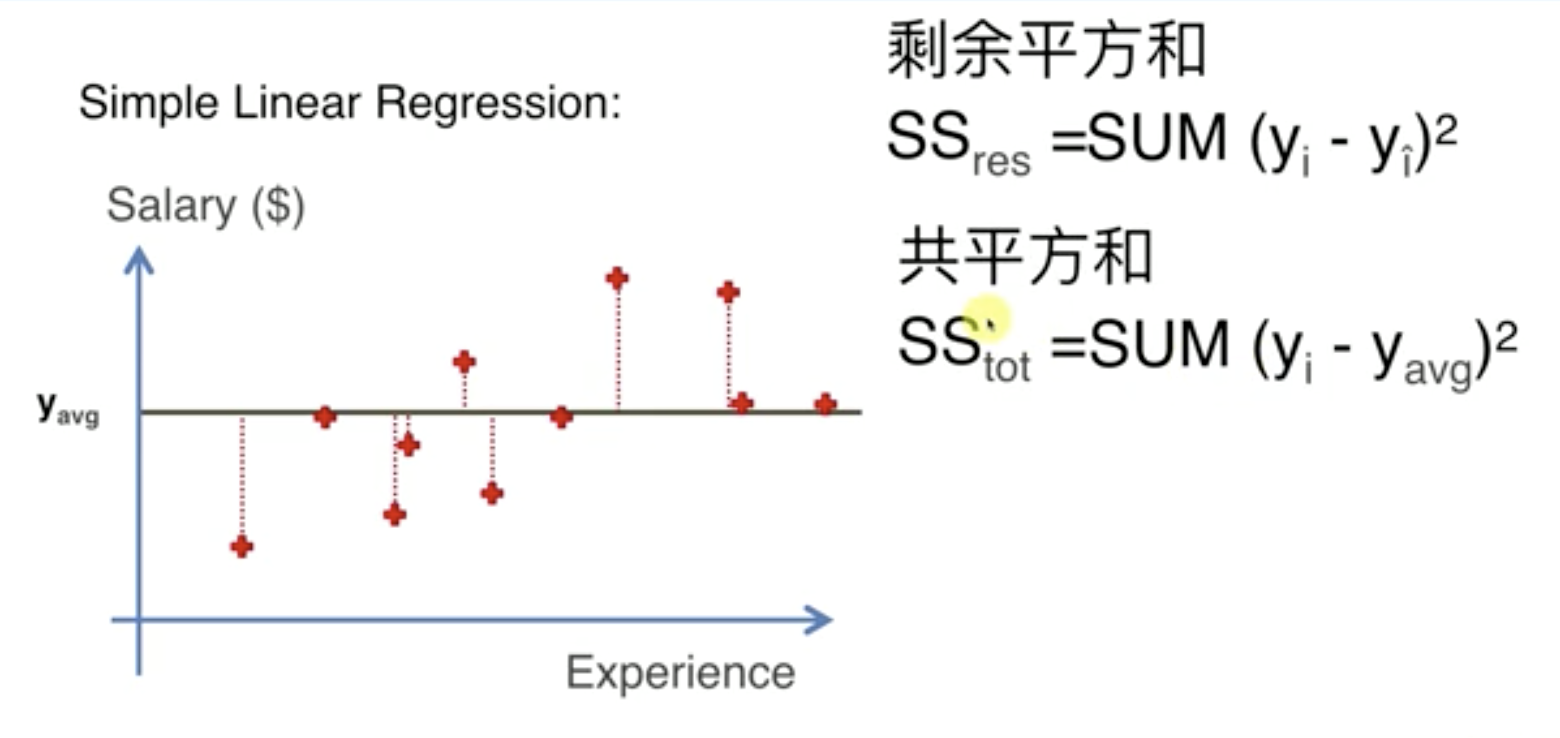## R平方R^2 = 1 – \frac{SS_{res}}{SS_{tot}}

Adj R^2 = 1 – (1 – R^2) * \frac{n-1}{n-p-1}